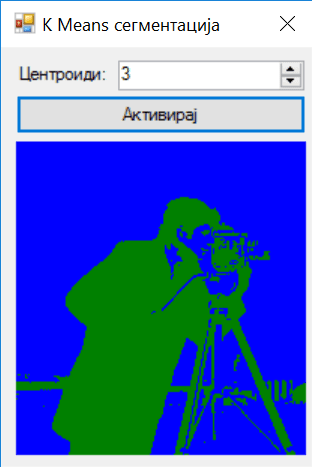# Image Segmentation with K-Means in C#As part of the K-Means tutorial series, we will take a look at the Image Segmentation problem.

## What is Image Segmentation?

Image Segmentation is the process of partitioning a digital image into multiple segments. The goal of segmentation is to simplify and/or change the representation of an image into something more meaningful or easier to analyze (source Wikipedia).

To put things into perspective, lets look at the famous cameraman image.

The goal for this project would be to segment the image into two important parts. That would be the foreground (the person on the image) and background (everything else). This information might be useful if we want to understand what is happening on the image.

The technique explained here, will allow us to create a pixel-wise mask for each object on the image. Even thought this tutorial is very basic, it is a good introduction into the problem. Next we will build more advanced algorithms using Neural Networks.

In this project we will use K-Means algorithm to classify similar pixels into k number of clusters.

## Creating the data set

The data set is represented by a vector with three elements. Since we are looking to group similar pixels in the image, we will need to use the pixel intensity for each channel. So the input vector would look something like this:

Input Vector = { Red, Green, Blue}

## Segmentation process

Since we are using K-Means we need to choose how many centroids we would like to create. This actually translates into how many objects we would like to segment out of the image. But keep in mind that this is not a very accurate segmentation algorithm. All that K-Means do is classify similar RGB vector points. So similar pixels will be grouped together.

If you are not familiar with K-Means clustering, you can start reading here. You can also take a look at other projects on my blog as well:

## How does it work?

It’s pretty simple actually. Like we said, we first choose how many centroids we would like to use for K-Means. Then we start processing the image. We take every single pixel RGB values and create a vector.

``````Color c = bmpImage.GetPixel(i, j);
double[] pixelArray = new double[] { c.R, c.G, c.B };``````

Next, those vectors are entered into a dataset. That dataset is fed into the K-Means algorithm. K-Means will try to classify each and every vector into one of the two selected centroids. This means that similar pixels will belong to same group.

This project also color codes the image. Color coding is also pretty simple. To each K-Means centroid you can assign a color. Next what you would like to do is, classify each individual pixel into one of the chosen groups/centroids.

``````    Color c = bmpImage.GetPixel(i, j);
double[] pixelArray = new double[] { c.R, c.G, c.B };

double minDistance = Double.MaxValue;
Centroid centroidWinner = null;
foreach (Centroid centroid in centroids)
{
double distance = objDistance.Run(pixelArray, centroid.Array);
if (distance < minDistance)
{
minDistance = distance;
centroidWinner = centroid;
}
}
bmpNew.SetPixel(i, j, centroidWinner.CentroidColor);
}``````

In order to get things more clear, download and browse the solution. The goal for this post is to introduce the image segmentation problem and solve it using the most easy technique available.

We will add a lot more to this. In the next couple of post we will try more advanced algorithms and we will eventually end up using state of the art Neural Networks.